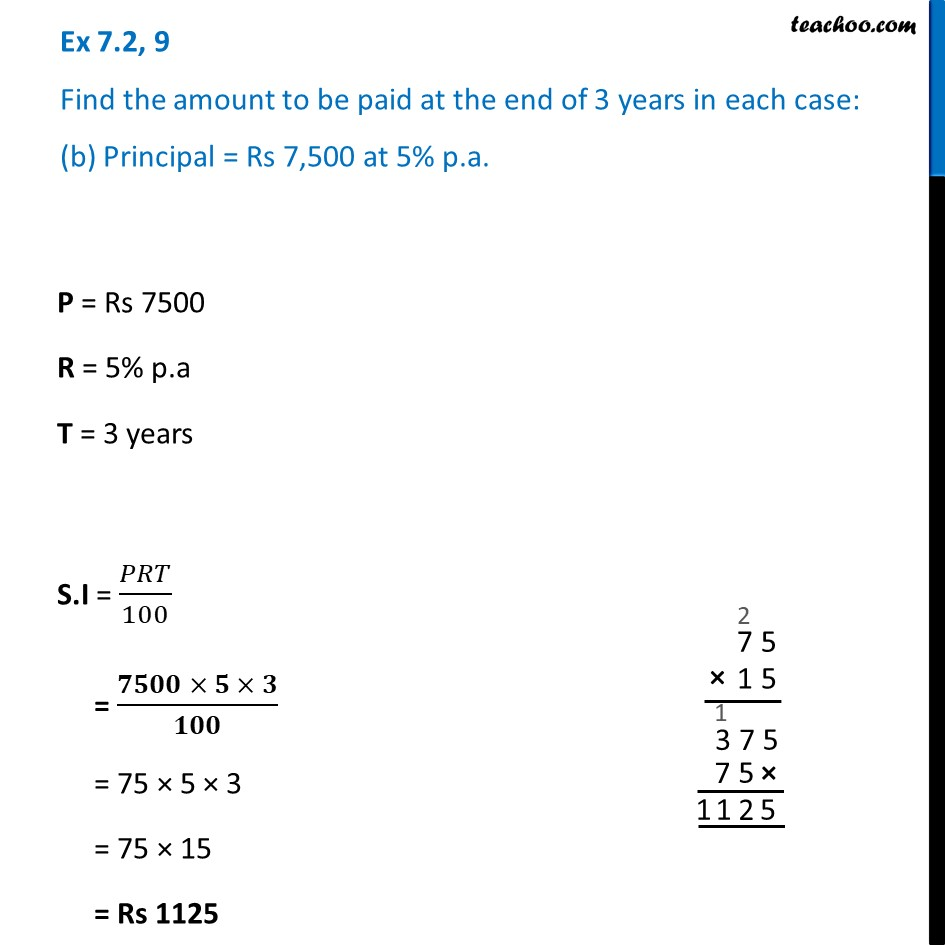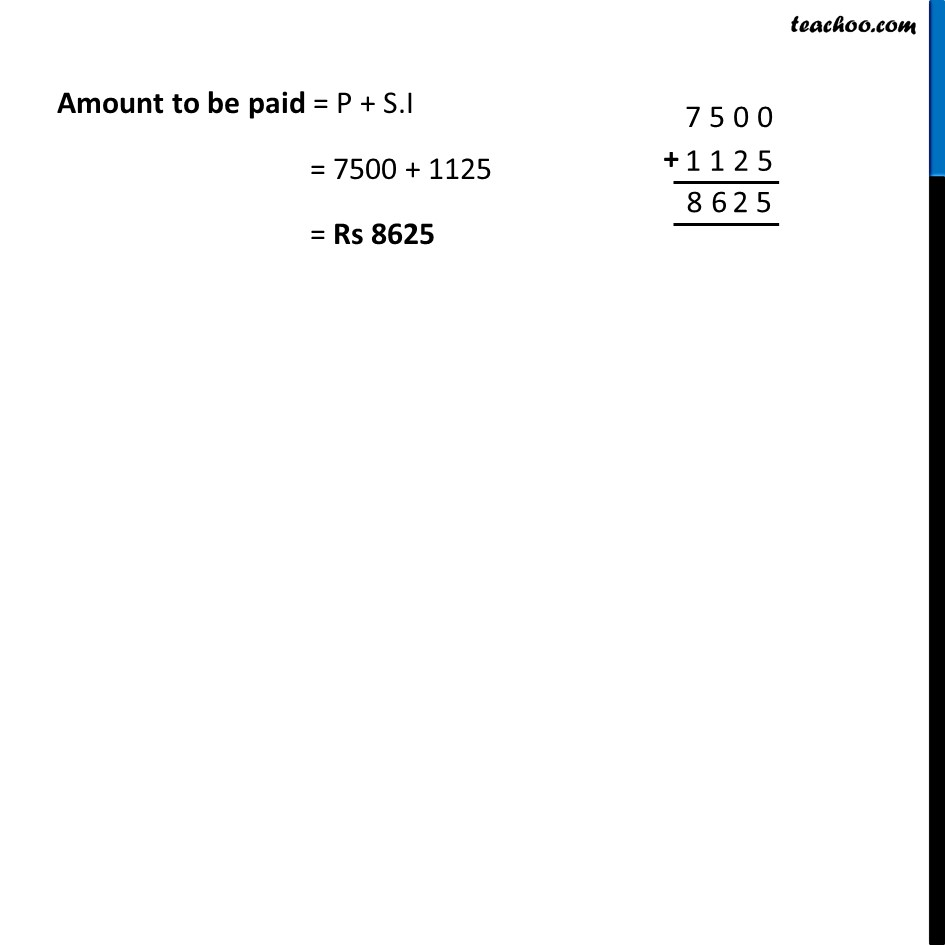Ex 7.2

Chapter 7 Class 7 Comparing Quantities
Serial order wiseLearn in your speed, with individual attention - Teachoo Maths 1-on-1 Class

### Transcript

Ex 7.2, 9 Find the amount to be paid at the end of 3 years in each case: (b) Principal = Rs 7,500 at 5% p.a. P = Rs 7500 R = 5% p.a T = 3 years S.I = 𝑃𝑅𝑇/100 = (𝟕𝟓𝟎𝟎 × 𝟓 × 𝟑)/𝟏𝟎𝟎 = 75 × 5 × 3 = 75 × 15 = Rs 1125 Amount to be paid = P + S.I = 7500 + 1125 = Rs 8625